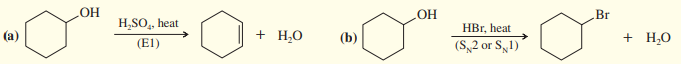×
Get Full Access to Organic Chemistry - 8 Edition - Chapter 6 - Problem 62sp
Get Full Access to Organic Chemistry - 8 Edition - Chapter 6 - Problem 62sp

×

# Protonation converts the hydroxyl group of an alcohol to aISBN: 9780321768414 33

## Solution for problem 62SP Chapter 6

Organic Chemistry | 8th Edition

• Textbook Solutions
• 2901 Step-by-step solutions solved by professors and subject experts
• Get 24/7 help from StudySoup virtual teaching assistantsOrganic Chemistry | 8th Edition

4 5 1 368 Reviews
31
0
Problem 62SP

Protonation converts the hydroxyl group of an alcohol to a good leaving group. Suggest a mechanism for each reaction.Equation Transcription:Text Transcription:

OH

(E1) \rightarrow H2SO4, heat+ H2O

OH

(SN2 or SN1) \rightarrow HBr, heat                 - Br + H2O

Step-by-Step Solution:
Step 1 of 3

Solution 62SP

1. The mechanism of reaction is given below -When cyclohexanol is protonated in presence of acid () it givescarbonated. In second step proton is lost to give cyclohexene.When Br- attack directly then -OH is substituted by -Br to give bromocyclohexane by2 mechanism.

Bromocyclohexane can also obtained bymechanism. In this firs carbocation is formed by protonation of -OH group. Then Br- attacks on carbonation to give bromocyclohexaneStep 2 of 3

Step 3 of 3

##### ISBN: 9780321768414

The full step-by-step solution to problem: 62SP from chapter: 6 was answered by , our top Chemistry solution expert on 05/06/17, 06:41PM. This textbook survival guide was created for the textbook: Organic Chemistry, edition: 8. Organic Chemistry was written by and is associated to the ISBN: 9780321768414. This full solution covers the following key subjects: Group, leaving, good, alcohol, hydroxyl. This expansive textbook survival guide covers 25 chapters, and 1336 solutions. Since the solution to 62SP from 6 chapter was answered, more than 342 students have viewed the full step-by-step answer. The answer to “?Protonation converts the hydroxyl group of an alcohol to a good leaving group. Suggest a mechanism for each reaction. Equation Transcription:Text Transcription:OH(E1) \rightarrow H2SO4, heat+ H2OOH(SN2 or SN1) \rightarrow HBr, heat - Br + H2O” is broken down into a number of easy to follow steps, and 35 words.

## Discover and learn what students are asking

Statistics: Informed Decisions Using Data : The Normal Approximation to the Binomial Probability Distribution
?Suppose X is a binomial random variable. To approximate P(3 ? X ? 10), compute _________ .

Statistics: Informed Decisions Using Data : Estimating the Value of a Parameter
?What does the 95% represent in a 95% confidence interval?

Statistics: Informed Decisions Using Data : Comparing Three or More Means (One-Way Analysis of Variance)
?Which Delivery Method Is Best? At a community college, the mathematics department has been experimenting with four different delivery mechanisms for c

Statistics: Informed Decisions Using Data : Inference about Measures of Central Tendency
?Locate the efficiency of the sign test in Section 15.1 for both large samples and small samples. What do these values indicate?

Statistics: Informed Decisions Using Data : Inference about the Difference between Two Medians: Dependent Samples
?Effects of Exercise A physical therapist wishes to learn whether an exercise program increases flexibility. She measures the flexibility (in inches) o

Unlock Textbook Solution